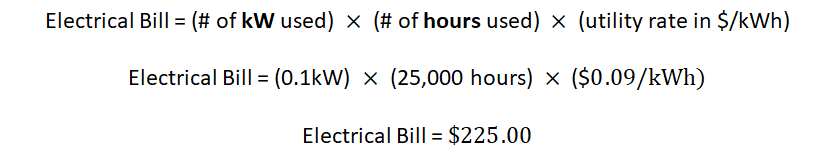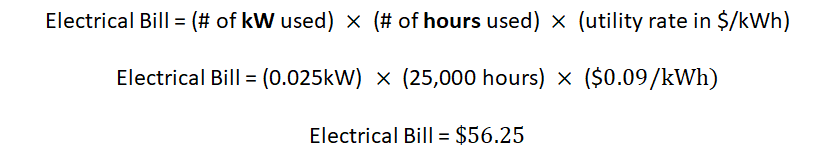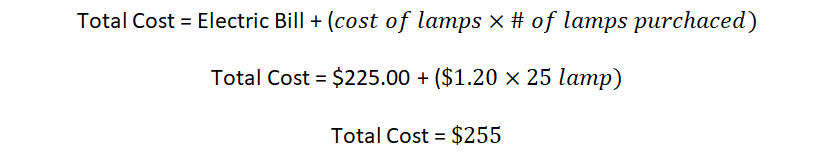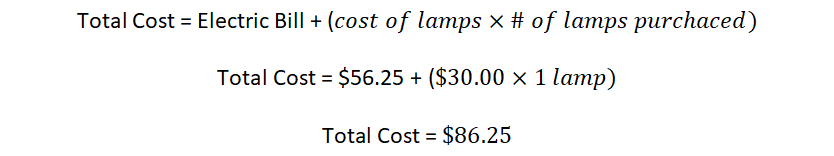# 23 Energy Cost Example 2 – 25,000 hours

Aaron Lee

Now we will compare the same lamps over a 25,000 hour period. Recall that the 100-watt incandescent lamp costs \$1.20 to purchase and has an average lamp life of 1000 hours, while the 25-watt LED lamp costs \$30.00 and lasts 25,000 hours. If the cost of energy is \$0.09 per kilowatt-hour.

Determine the electrical cost of running each load for 25,000 hours.

Incandescent electrical cost:LED electrical cost:Factoring in the cost of the lamps and we can determine the total cost of each lighting system for 25,000 hours.

Incandescent total cost:LED total cost:While the LED has a high initial cost of \$30.00, due to its extended lifespan it actually costs just as much as the incandescent lamps since we would need approximately 25 incandescent lamps for the 25,000 hour period. (25 x \$1.20 = \$30.00).

The LEDs massive savings in energy use and extended lifespan make it the preferred choice for lighting installations.